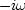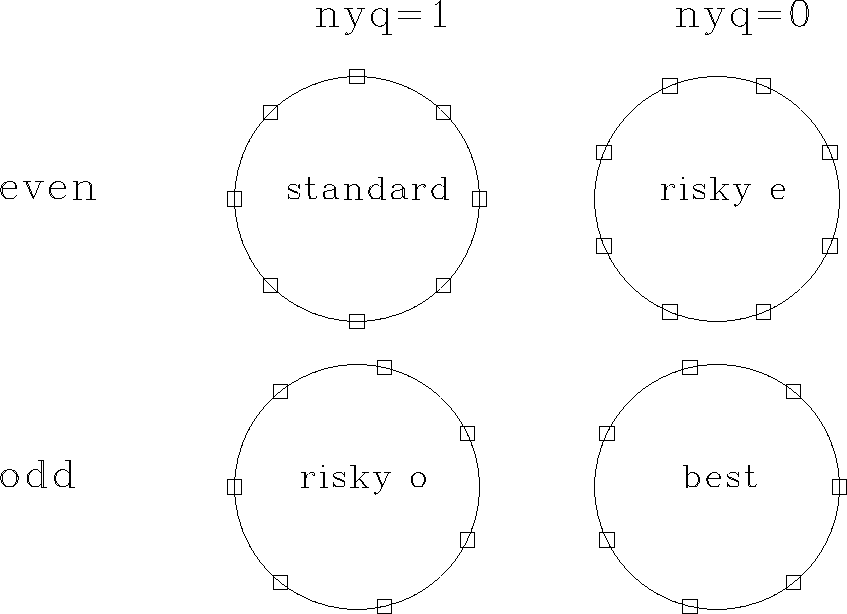Next: FT by Z-transform Up: INVERTIBLE SLOW FT PROGRAM Previous: The slow FT code

## Truncation problems

When real signals are transformed to the frequency domain, manipulated there, and then transformed back to the time domain, they will no longer be completely real. There will be a tiny noise in the imaginary part due to numerical roundoff. The size of the imaginary part, theoretically zero, is typically about 10-6 of the real part. This is also about the size of the error on the real part of a signal after inverse transform. It is almost always much smaller than experimental errors and is of little consequence. As a check, I viewed these near-zero imaginary parts, but I do not show them here.

A more serious error is a relative one of about 1/N on an N-point signal. This arises from insufficient care in numerical analysis, especially associated with the ends of the time or frequency axis. To show end effects, I will print some numbers resulting from processing very short signals with slowft(). Below I show first the result that a transform followed by an inverse transform gives the original signal. I display this for both even and odd lengths of data, and for the two Nyquist arrangements as well.

Inversion: You should see (2,1,0,0)
nyq=0   2.00   1.00   0.00   0.00
nyq=1   2.00   1.00   0.00   0.00
nyq=0   2.00   1.00   0.00   0.00   0.00
nyq=1   2.00   1.00   0.00   0.00   0.00


Second, I display the result of a test of the convolution theorem by convolving (2,1) with (1,-1). We see that the scale factor varies with the data size because we are using the energy-conserving FT, instead of equations (3) and (4). No problems yet.

Convolution theorem: Proportional to (0,2,-1,-1,0,0,0,0)
nyq=0   0.00   0.89  -0.45  -0.45   0.00
nyq=1   0.00   0.89  -0.45  -0.45   0.00
nyq=0   0.00   0.82  -0.41  -0.41   0.00   0.00
nyq=1   0.00   0.82  -0.41  -0.41   0.00   0.00


The third test is delaying a signal by two samples using ftlagslow(). Here the interesting question is what will happen at the ends of the data sample. Sometimes what shifts off one end shifts back in the other end: then the signal space is like the perimeter of a circle. Surprisingly, another aggravating possibility exists. What shifts off one end can return in the other end with opposite polarity. When this happens, a figure like 4 looks much rougher because of the discontinuity at the ends. Even if there is no physical signal at the ends, the ripple we see in Figure 4 reaches the ends and worsens. (Recall that nyq=1 means the Nyquist frequency is included in the spectrum, and that nyq=0 means it is interlaced.)

Delay tests:
In               11.0   12.0   13.0   14.0   15.0   16.0   17.0
Out  n=7 nyq=0   16.0   17.0   11.0   12.0   13.0   14.0   15.0
Out  n=7 nyq=1  -16.0  -17.0   11.0   12.0   13.0   14.0   15.0
Out  n=6 nyq=0  -15.0  -16.0   11.0   12.0   13.0   14.0
Out  n=6 nyq=1   15.0   16.0   11.0   12.0   13.0   14.0


The fourth test is to do a time derivative in the frequency domain with subroutine ftderivslow(). Here we do not have quite so clear an idea of what to expect. The natural position for a time derivative is to interlace the original data points. When we make the time derivative by multiplying in the frequency domain by, however, the derivative does not interlace the original mesh, but is on the same mesh. The time derivative of the small pulse we see here is the expected doublet aligned on the original mesh, and it has some unexpected high-frequency ripple that drops off slowly. The ripple resembles that on a pulse shifted half a mesh point, as in Figure 4. It happens that this rippling signal is an accurate representation of the derivative in many examples where such mesh alignment is needed, so (as with time shift) the ripple is worth having. Here again, we notice that there is an unfortunate transient on the ends of the data on two of the tests. But in two of the four tests below, the transient is so huge that it overwhelms the derivative of the small pulse in the middle of the signal.

Derivative tests:
In              10.0  10.0  10.0  10.0  12.0  10.0  10.0  10.0  10.0
Out  n=9 nyq=0  -0.7   0.8  -1.1   2.0   0.0  -2.0   1.1  -0.8   0.7
Out  n=9 nyq=1  13.5  -5.1   2.0   0.7   0.0  -0.7  -2.0   5.1 -13.5
Out  n=8 nyq=0  13.2  -5.7   3.5  -1.9   3.9  -6.6   7.6 -14.8
Out  n=8 nyq=1   0.0   0.3  -0.8   1.9   0.0  -1.9   0.8  -0.3


Examining all the tests, we conclude that if the data has an even number of points, it is best to include the Nyquist frequency in the frequency-domain representation. If the data has an odd number of points, it is better to exclude the Nyquist frequency by interlacing it. A more positive way of summarizing our results is that the zero frequency should always be present. Given this conclusion, the next question is whether we should choose to use an even or an odd number of points.

The disadvantage of an even number of data values is that the programs that do frequency-domain manipulations will always need to handle Nyquist as a special case. The value at the Nyquist frequency must be handled as if half of it were at plus Nyquist and the other half at minus Nyquist. The Nyquist aggravation will get worse in two dimensions, where we have corners as well as edges.

 circeval Figure 4 Evaluation of various arrangements of frequencies.Figure 5 reproduces the four arrangements in Figure 1 along with a one-word summary of the suitability of each arrangement: standard" for the standard arrangement, risky" for arrangements that have end effects that are likely to be undesirable, and best" for the arrangement that involves no risky end effects and no pesky Nyquist frequency.

Later in this chapter we will see the importance of using a fast FT program--one which is orders of magnitude faster than slowft(). Unfortunately, among fast FT programs, I could not find one for an odd-length transform that is suitable for printing here, since odd-length FT programs seem to be many pages in length. So further applications in this book will use the even-length program. As a result, we will always need to fuss with the Nyquist frequency, making use of the frequency arrangement labeled standard" and not that labeled best."

 A discrete Fourier-transform program designed for an odd number of points would make applications somewhat simpler. Alas, there seems to be no program for odd-length transforms that is both simple and fast.Next: FT by Z-transform Up: INVERTIBLE SLOW FT PROGRAM Previous: The slow FT code
Stanford Exploration Project
10/21/1998